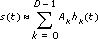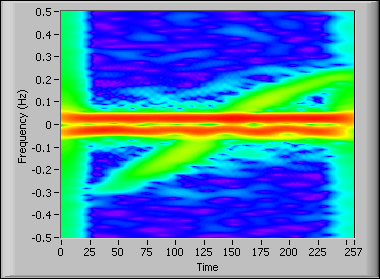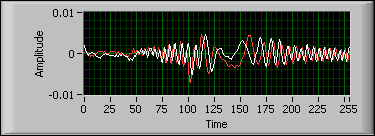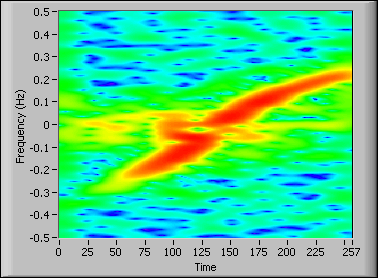LabVIEW 2014 Advanced Signal Processing Toolkit Help

Edition Date: June 2014

Part Number: 372656C-01

»View Product InfoDownload Help (Windows Only)

You can use the inverse Fourier transform and the discrete Gabor expansion to express signals as a linear combination of a series of elementary functions. For example, the inverse discrete Fourier transform expresses a signal as the linear combination of sinusoids in which the weight of each elementary sinusoid is the corresponding discrete Fourier coefficient. The discrete Gabor expansion expresses a signal as the linear combination of windowed sinusoids with Gabor transform coefficients.

Like the discrete Gabor transform, the adaptive expansion expresses a signal as a linear combination of Gaussian-windowed, linear-chirp functions called chirplets.

In the following equation, the adaptive expansion represents a signal, s(t), as the linear combination of a series elementary functions, hk(t), approximately:where Ak is the corresponding complex amplitude of hk(t), and D specifies the number of the elementary functions. The adaptive transform computes the complex amplitudes, Ak.

The adaptive transform, also called the adaptive chirplet decomposition, computes the weight for each elementary chirplet. Because the sinusoid function is a subset of the chirplet, the adaptive transform is more powerful than the Gabor transform but generally requires more computing time. The adaptive transform and the adaptive expansion are unique to the LabVIEW Time Frequency Analysis Tools.

Unlike the discrete Gabor expansion and discrete Gabor transform, the elementary functions of the adaptive expansion are time-varying signals themselves with spectral content that changes linearly over time. The time centers and the frequency centers of the chirplets are not limited to a grid in the time-frequency plane, and they can be any real value. Also, the window lengths of all the chirplets do not need to be the same. Therefore, the adaptive expansion can have a finer time-frequency resolution and express time-varying signals more accurately.

## Matching Pursuit Method

The matching pursuit method is a commonly used implementation of the adaptive transform that uses a set of elementary functions called a dictionary. The dictionary size in the matching pursuit algorithm determines the speed and accuracy of the resulting analysis. A small dictionary requires less computing time but has poorer accuracy. A large dictionary results in better accuracy but requires more computing time. Refer to the book Introduction to Time-Frequency and Wavelet Transforms for more information about the matching pursuit method.

The TFA Adaptive Transform VI provides an implementation of the adaptive transform that is more efficient and accurate than the matching pursuit method. This implementation uses the matching pursuit method with a small dictionary size as a coarse estimation step and then follows with a refinement step to achieve an accurate estimation. The small dictionary size and refinement step make the adaptive transform in the Time Frequency Analysis Tools more efficient and accurate.

The adaptive transform is used widely in applications, such as radar and sonar signal processing, that need to accurately estimate parameters of signals with time-variant spectra, especially signals that contain chirplets. After you detect the adaptive chirplets in a signal, you also can use adaptive chirplets to improve the performance of some pattern-recognition applications.

The following figure shows the typical steps to create an adaptive transform and adaptive expansion application.Usually, you use the adaptive transform to compute the magnitudes and parameters of chirplets, select the chirplets that you need for the application, and then apply the adaptive expansion to reconstruct the signal. In some applications, you might use the parameters of chirplets to make a decision before the expansion.

The white line in the following figure represents the real parts of a complex-valued signal from a pulse radar receiver, and the red line in this figure represents the imaginary parts of the signal.Though not apparent in the previous figure, this signal contains three signals—the target signature signal and two instances of strong interference from sea clutter, which is the radar signal reflected off of surface sea waves. The interference signal is much stronger than the target signature signal so you cannot see the target signature in the time-domain representation in the previous figure. The goal of this application is to remove the interference signal and extract the target signature signal.

Because the target signature signal is a time-varying chirp signal, first observe the time-frequency representation of the signal before selecting a signal processing method to use. The following figure shows the STFT magnitude spectrum of the example signal.The color corresponds to the magnitude of the STFT coefficients. The color spectrum from red to blue corresponds to the magnitude from maximum to minimum. The red stripes close to 0 Hz are the sea clutter interference. The green stripe running diagonally across the interference signal is the target signature signal. Notice that the interference signal is stronger than the target signature signal and that the interference and the target signature overlap in the time-frequency plane. A conventional time-domain fixed filter is not sufficient for separating these components. Because the spectral peak of the target signature changes over time and the spectral bandwidths of the interference vary over time, you can use the TFA Adaptive Transform VI to decompose the signal into a linear combination of chirplets. Next, you can remove the resulting chirplets with a chirp rate close to zero and a frequency less than 0.02 Hz in this example. Then you can use the TFA Adaptive Expansion VI to reconstruct the target signature, as shown in the following figure.Compared to the interference signal in the figure of the complex signal from the pulse radar receiver, the sea clutter signal in the previous figure has been suppressed up to 20 dB. The following figure shows the STFT magnitude spectrum of the extracted target signature with the interference substantially reduced.Refer to the Chirplet TDR VI in the labview\examples\Time Frequency Analysis\TFAApplications directory for an example of using the TFA Adaptive Transform VI and the TFA Adaptive Expansion VI.

If the signal you want to analyze using any linear time-frequency method contains a large constant offset or is non-negative, a single line in the vicinity of 0 Hz dominates the resulting time-dependent spectrum. In these situations, you might not be able to identify more interesting frequency patterns. To suppress the constant offset component, you can apply certain types of preprocessing, but the detrending methods for removing the constant-offset components depend on the application. No general method works in all cases. Common techniques of detrending include lowpass filtering and curve fitting. However, another technique is the wavelet transform.